I.2.2

2/2/2021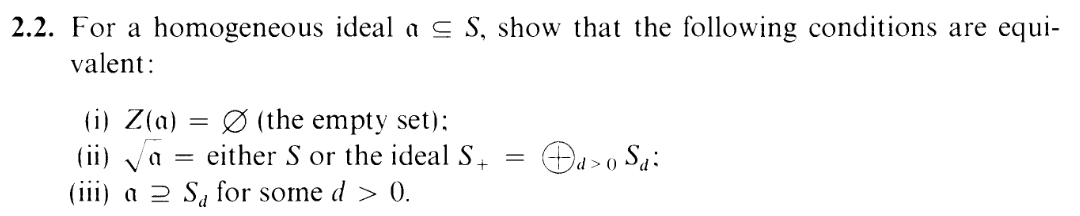This post is mostly straight math. Feel free to skip this post if you only like squiggly math.

SOME NOTES TO MYSELF BEFORE BEGINNING:
I covered some of the cases of Z(α) = in the previous post. Note that, in (ii), the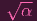= S case DOESN'T break the homogenous Nullstellensatz, whereas the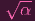= S + COULD break the homogenous Nullstellensatz.

Also, in the previous post, I supposed that the degree of 0 in S = k[x0,x1,,xn] ought to be 0. Oddly, it appears that this isn't the case. It appears that 0 transcends degree. See condition (ii) in this exercise. The ideal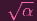can be equal to S+, which contains NO zero degree elements. And yet, since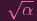is an ideal, we must have 0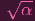i.e. 0 S+. So 0 is in the positive degree part of S. However. S0 itself is the same as k, and certainly 0 k. so 0 S0. So it's in the zero degree part of S as well. SO, basically, you can't put a degree on 0. I'll keep that in mind, I guess.

OKAY NOTES FIN. LET'S BEGIN.

(i)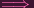(ii):

Let f S be homogenous positive degree. Then vacuously P Z(α) : f(P) = 0 (since (i) says Z(α) = ). By the homgenous Nullstellensatz (2.1), fq α for some q > 0 i.e. f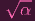.

Thus, we've established that S+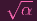.

CASE 1: 1 α
Then α = S.

CASE 2: 1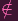α
Then certainly 1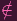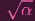(else 1q = 1 α, a contradiction). But if 1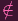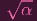then c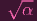for any nonzero constant c k (otherwise we'd have c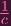= 1 α, a contradiction). I.e. c S0 : c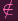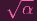. So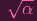must be contained in S+. I.e.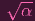S +.

DONE.

(ii)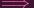(iii):

CASE 1: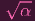= S
Then 1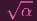, which means that 1q α for some q, but this just means that 1 alpha i.e. α = S. But S Sd. CASE 1 DONE.

CASE 2: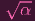= S +:
Suppose THIS were true: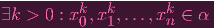(1)

(we're working in Pn by the way lol)
(also, shouldn't have used k cause that usually refers to the field but fuck it too late lol)
Then for d = k(n + 1), consider Sd.
Let cx0k0x1k1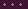xnkn be an arbitrary term of Sd.
We have to have k0 + k1 +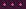+ kn = d, which means that we have to have ki >=k for at least one i. Assume without loss of generality that i = 0. Then we can rewrite that arbitrary term by factoring out an x0k:
x0k(cx0k0-kx1k1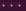xnkn)
But this guy is actually in α, since x0k α (by (1)) and x0k f α for any f S (since α is an ideal). So we basically showed that an arbitrary element of S0 is in α. I.e. we showed S0 α.
To finish off case 2, we just need to show that (1) is true. Since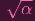= S by assumption, we must have x0,,xn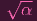. I.e. for each i = 0,,n, we have ximi α for some mi > 0. But then certainly xim0m1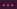mi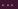mn α. (e.g. (x0m0)m1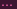mn α, etc.).
CASE 2 DONE.

(iii)(i):

We're given α Sd. Taking Z() of both sides, we reverse the inclusion, yielding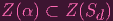(2)

But since x0d,x1d,,xnd Sd, a point P would have to be all 0 in order to be 0 at all of these (since k is an integral domain!) and this can't happen in Pn, so we actually have Z(Sd) = . So by (2), Z(α) ⊂∅ i.e. Z(α) = .